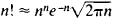# Stirling's Formula

Also found in: Dictionary, Wikipedia.
Related to Stirling's Formula: Gamma function, Wolfram Alpha

## Stirling's formula

[′stir·liŋz ‚fȯr·myə·lə]
(mathematics)
The expression (n / e)n√(2π n) is asymptotic to factorial n; that is, the limit as n goes to ∞ of their ratio is 1.
McGraw-Hill Dictionary of Scientific & Technical Terms, 6E, Copyright © 2003 by The McGraw-Hill Companies, Inc.
The following article is from The Great Soviet Encyclopedia (1979). It might be outdated or ideologically biased.

## Stirling’s Formula

a formula giving the approximate value of the product of the first n natural numbers 1 × 2 × . . . × n = n! when n is large. In other words, the formula provides an approximation of the factorial of n. Stirling’s formula was discovered by J. Stirling, who published it in 1730. He did not, however, provide an estimate of the error. The formula establishes the approximate equalityHere, π = 3.14159 . . ., and e = 2.71828 ... is the base of the natural logarithms.

When n! is calculated by means of this formula, the relative error is less than ewln 1 and thus approaches 0 as n increases without bound. When n = 10, for example, the formula yields n! = 3,598,700, whereas the exact value of 10! is 3,628,800. In this case, the relative error is less than 1 percent.

Stirling’s formula has numerous applications in probability theory and mathematical statistics.

### REFERENCE

Fikhtengol’ts, G. M. Kurs differentsial’nogo i integral’nogo ischisleniia, 7th ed., vol. 2. Moscow, 1969.